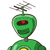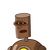# What is the median of the following data set? {6, 3, 9, 1, 7}

What is the median of the following data set?

{6, 3, 9, 1, 7}

### 2 thoughts on “What is the median of the following data set?<br /><br /> {6, 3, 9, 1, 7}”

1.Step-by-step explanation:

Hey mate!

Since, the no. only terms in the given data is odd.

Therefore, the median is( N+1)/2 th value that is 5+1)/2th term = 3rd term = 9 .

2.Step-by-step explanation:

To find a median

first arrange in ascending order

1, 3, 6, 7, 9

Now,

median= n+1 /2 (observation)

= 5+1/2= 6/2= 3

so the median is the 3rd observation= 6